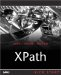Subtracting xs:dateTime Values

Subtracting xs:dateTime Values

The final date/time functions in this chapter involve subtracting xs:dateTime values. We saw earlier that there are various operators that take care of subtraction for the date/time types, but with these functions you can specify if you want the result as an xdt:yearMonthDuration value or an xdt:dayTimeDuration value:

• fn:subtract-dateTimes-yielding-yearMonthDuration returns the difference between two xs:dateTime values as an xdt:yearMonthDuration value.

• fn:subtract-dateTimes-yielding-dayTimeDuration returns the difference between two xs:dateTime values as an xdt:dayTimeDuration value.

The fn:subtract-dateTimes-yielding-yearMonthDuration Function

As you can gather from its name , this function returns an xdt:yearMonthDuration value when you subtract two xs:dateTime values. Here's how you use it:

fn:subtract-dateTimes-yielding-yearMonthDuration(  \$srcval1  as xs:dateTime,  \$srcval2  as xs:dateTime) as xdt:yearMonthDuration

The return value is an xdt:yearMonthDuration value containing the difference between \$srcval1 and \$srcval2 .

For example, this expression returns an xdt:yearMonthDuration value holding the value 11 months:

fn:subtract-dateTimes-yielding-yearMonthDuration(xs:dateTime("2004-01-30T19:48:00"),     xs:dateTime("2003-02-28T12:00:00"))

The fn:subtract-dateTimes-yielding-dayTimeDuration Function

This function returns the difference between two xs:dateTime values as an xdt:dayTimeDuration value:

fn:subtract-dateTimes-yielding-dayTimeDuration(\$srcval1 as xs:dateTime,     \$srcval2 as xs:dateTime) as xdt:dayTimeDuration

The return value is an xdt:dayTimeDuration value that contains the difference between \$srcval1 and \$srcval2 as an xdt:dayTimeDuration value.

For example, this expression returns an xdt:dayTimeDuration value corresponding to 1 day, 3 hours, 22 minutes, and 2 seconds:

fn:subtract-dateTimes-yielding-dayTimeDuration(xs:dateTime("2004-04-09T04:22:07"),     xs:dateTime("2004-04-08T01:00:05"))XPath Kick Start: Navigating XML with XPath 1.0 and 2.0
ISBN: 0672324113
EAN: 2147483647
Year: 2002
Pages: 131

Similar book on Amazon